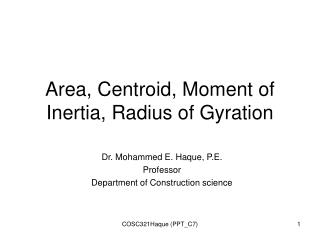DownloadDownload PresentationArea, Centroid, Moment of Inertia, Radius of Gyration

# Area, Centroid, Moment of Inertia, Radius of Gyration

Télécharger la présentation## Area, Centroid, Moment of Inertia, Radius of Gyration

- - - - - - - - - - - - - - - - - - - - - - - - - - - E N D - - - - - - - - - - - - - - - - - - - - - - - - - - -
##### Presentation Transcript

1. Area, Centroid, Moment of Inertia, Radius of Gyration Dr. Mohammed E. Haque, P.E. Professor Department of Construction science COSC321Haque (PPT_C7)

2. y A = b h Ix = b h3 /12 Iy = h b3 /12 x h b Area, Moment of Inertia Centroid COSC321Haque (PPT_C7)

3. Area, Moment of Inertia y A = 0.5 b h Ix = b h3 /36 Iy = h b3 /36 h x Centroid h/3 b/3 b COSC321Haque (PPT_C7)

4. Area, Moment of Inertia Y Centroid X R A = π R2 Ix = Iy = π R4 /64 COSC321Haque (PPT_C7)

5. Radius of Gyration rx =(Ix /A) ry =(Iy /A) COSC321Haque (PPT_C7)

6. Centroid b/2 A = b h h/2 h Ixc = b h3 /12 dy Ix-x = Ixc + A dy2 = b h3 /12 + b h dy2 b x x Moment of Inertia about an axis parallel to centroidal axis COSC321Haque (PPT_C7)

7. 20’-0” Y 4’-0” 4’-0” 10’-0” 4’-0” 7’-0” 3’-0” X 3’-0” 14’-0” 3’-0” Area and Centroid Q1: A pre-cast concrete wall panel as shown in fig. Determine (a) Wall Area (b) Centroid (x and y axes referenced from the lower left corner). COSC321Haque (PPT_C7)

8. A = 163 Sqft X = 1713.5 /163 = 10.512 ft Y = 846.5 /163 = 5.193 ft COSC321Haque (PPT_C7)

9. y 2” X Y 3” 5” 3” 2” x y 2” 1 5” 3” 3” 2” 2 x Q2: Determine (a) Area (b) Centroid (c ) Moment of Inertia about x and y axes COSC321Haque (PPT_C7)

10. X = 104 /26 = 4 in Y = 61 /26 = 2.346 in (a) Area; (b) Centroid • AREA, A = 26 Sqin. • (b) COSC321Haque (PPT_C7)

11. (c ) Moment of Inertia about the centroidal axes Ixcg = 26.167 + 75.384 = 101.55 in4 Iycg = 88.667 + 0 = 88.667 in4 COSC321Haque (PPT_C7)

12. Y 1” X 4” 1” 2” 2” 2” • Q3: Determine • Area • Moment of Inertia, Ixc, Iyc • Radius of Gyration, rx, ry COSC321Haque (PPT_C7)

13. 1 3 Y 2 1” X 4” 1” 2” 2” 2” A= 20 in2 Ix = 11.667 + 75.0 = 86.667 in4 Iy = 38.667 + 0 = 38.667 in4 rx = (86.667/20) = 2.08 in ry = (38.667/20) = 1.39 in COSC321Haque (PPT_C7)

14. Y 1 1” X 4” 2 1” 2” 2” 2” • Q4: Determine • Area • Moment of Inertia, Ixc, Iyc • Radius of Gyration, rx, ry COSC321Haque (PPT_C7)

15. Y 1 1” X 4” 2 1” 2” 2” 2” A= 28 in2 Ix = 97.333 in4 Iy = 105.333 in4 rx = (97.333/28) = 1.86 in ry = (105.333/28) = 1.94 in COSC321Haque (PPT_C7)

16. Y 4” 1” X Y 2” 4” 1 4” 2 1” X 2” 4” • Q5: Determine • Area • Centroid • Moment of Inertia, Ixc, Iyc • Radius of Gyration, rx, ry COSC321Haque (PPT_C7)

17. X = 26 /14 = 1.86 in Y = 27 /14 = 1.93 in (a) Area; (b) Centroid • AREA, A = 14 Sqin. • (b) COSC321Haque (PPT_C7)

18. (c ) Moment of Inertia; (d) Radius of gyration Ix = 11.167 + 46.54 = 57.71 in4 Iy = 20.667 + 13.72 = 34.39 in4 rx = (57.71/14) = 2.03 in ry = (34.39/14) = 1.57 in COSC321Haque (PPT_C7)